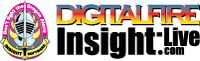•The secret to cool bodies and glazes is a lot of testing.
•The secret to know what to test is material and chemistry knowledge.
•The secret to learning from testing is documentation.
•The place to test, do the chemistry and document is an account at https://insight-live.com
•The place to get the knowledge is https://digitalfire.com

Sign-up at https://insight-live.com today.

# Cooley No. 2 Ball Clay

 Oxide Analysis Formula CaO 0.30% 0.017 MgO 0.40% 0.032 K2O 0.57% 0.020 Na2O 0.57% 0.030 TiO2 1.60% 0.065 Al2O3 31.50% 1.000 SiO2 53.30% 2.872 Fe2O3 1.60% 0.032 LOI 10.70 Oxide Weight 290.91 Formula Weight 325.77

## XML for Import into INSIGHT

<?xml version="1.0" encoding="UTF-8"?> <material name="Cooley No. 2 Ball Clay" descrip="" searchkey="" loi="0.00" casnumber="70694-09-6"> <oxides> <oxide symbol="CaO" name="Calcium Oxide, Calcia" status="" percent="0.300" tolerance=""/> <oxide symbol="MgO" name="Magnesium Oxide, Magnesia" status="" percent="0.400" tolerance=""/> <oxide symbol="K2O" name="Potassium Oxide" status="" percent="0.570" tolerance=""/> <oxide symbol="Na2O" name="Sodium Oxide, Soda" status="" percent="0.570" tolerance=""/> <oxide symbol="TiO2" name="Titanium Dioxide, Titania" status="" percent="1.600" tolerance=""/> <oxide symbol="Al2O3" name="Aluminum Oxide, Alumina" status="" percent="31.500" tolerance=""/> <oxide symbol="SiO2" name="Silicon Dioxide, Silica" status="" percent="53.300" tolerance=""/> <oxide symbol="Fe2O3" name="Iron Oxide, Ferric Oxide" status="" percent="1.600" tolerance=""/> </oxides> <volatiles> <volatile symbol="LOI" name="Loss on Ignition" percent="10.700" tolerance=""/> </volatiles> </material>